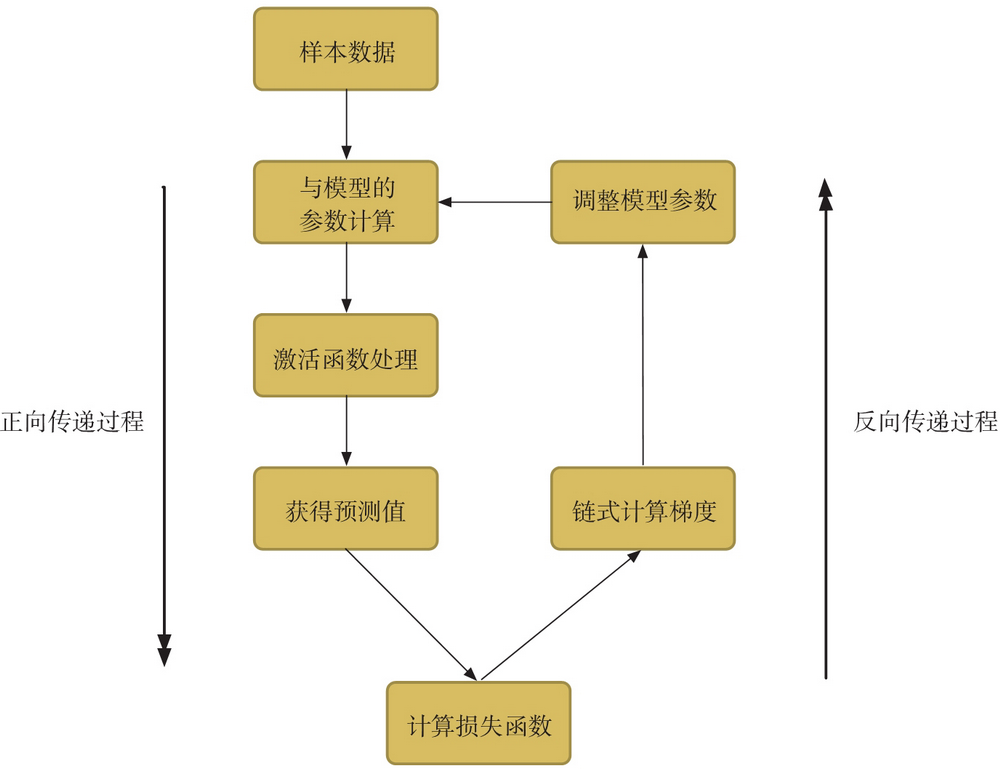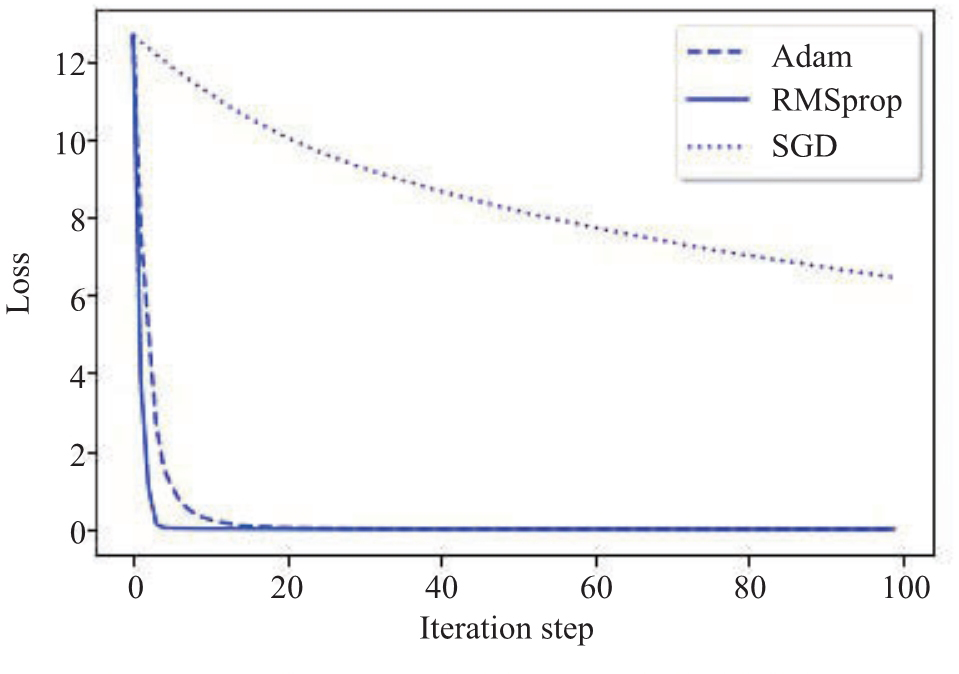# AI 安全之对抗样本入门 (4)：深度学习基础知识 1.1.4(反向传递与优化器)

$\triangledown f(x,y)=\left(\frac{\delta f(x,y)}{\delta x},\frac{\partial f(x,y)}{\partial y}\right)$

$x_{k+1}=x_k+\triangledown x_k^*\alpha$$x_{k+1}=x_k+\triangledown x_k^*\alpha$

$f(x)=x^2+2$

$\triangledown f(x)=\frac{\partial f(x)}{\partial x}=2x$

def demo():    import random    a=0.1    x=random.randint(1,10)    y = x * x + 2    index=1    while index < 100 and abs(y-2) > 0.01 :        y=x*x+2        print "batch={} x={} y={}".format(index,x,y)        x=x-2*x*a        index+=1

batch=14 x=0.329853488333 y=2.10880332377batch=15 x=0.263882790666 y=2.06963412721batch=16 x=0.211106232533 y=2.04456584141batch=17 x=0.168884986026 y=2.02852213851batch=18 x=0.135107988821 y=2.01825416864batch=19 x=0.108086391057 y=2.01168266793batch=20 x=0.0864691128455 y=2.00747690748

Keras 里面提供相应的工具返回 loss 函数关于 variables 的梯度，variables 为张量变量的列表，这里的 loss 函数即损失函数：

from keras import backend as Kk.gradients(loss, variables)

Keras 也提供了 function 用于实例化一个 Keras 函数，inputs 是输入张量的列表，其元素为占位符或张量变量，outputs 为输出张量的列表：

k.function(inputs, outputs, updates=[])

1. SGD

SGD 即随机梯度下降法，是最基础的优化方法。普通的训练方法需要重复不断地把整套数据放入神经网络中训练，这会消耗大量计算资源。SGD 则会把数据拆分后再分批不断地放入神经网络中来计算。每次使用批数据，虽然不能反映整体数据的情况，不过却在很大程度上加速了神经网络的训练过程，而且也不会丢失太多准确率1

1 https://ptorch.com/news/54.html

SGD 支持动量参数，支持学习衰减率，函数的定义如下：

keras.optimizers.SGD(lr=0.01, momentum=0.0, decay=0.0, nesterov=False)

• lr：学习率。
• momentum：动量参数。
• decay：每次更新后的学习率衰减值。
1. RMSprop

RMSprop 是面对递归神经网络时的一个良好选择，函数的定义如下：

keras.optimizers.RMSprop(lr=0.001, rho=0.9, epsilon=1e-06)

• lr：学习率。
• epsilon：大于或等于 0 的小浮点数，防止除 0 错误。

keras.optimizers.Adam(lr=0.001, beta_1=0.9, beta_2=0.999, epsilon=1e-08)

• lr：学习率。
• epsilon：大于或等于 0 的小浮点数，防止除 0 错误。

https://github.com/duoergun0729/adversarial_examples/code/1-case1-pytorch.ipynb

adam_original_loss = []sdg_original_loss=[]RMSprop_original_loss=[]epoch_range=[]

#获取计算设备，默认是 CPUdevice = torch.device("cuda" if torch.cuda.is_available() else "cpu")#图像加载以及预处理image_path="../picture/cropped_panda.jpg"orig = cv2.imread(image_path)[..., ::-1]orig = cv2.resize(orig, (224, 224))img = orig.copy().astype(np.float32)

mean = [0.485, 0.456, 0.406]std = [0.229, 0.224, 0.225]img /= 255.0img = (img - mean) / stdimg = img.transpose(2, 0, 1)img=np.expand_dims(img, axis=0)img = Variable(torch.from_numpy(img).to(device).float())

#使用预测模式主要影响 dropout 和 BN 层的行为model = models.alexnet(pretrained=True).to(device).eval()#获取分类标签label=np.argmax(model(img).data.cpu().numpy())print("label={}".format(label))#图像数据梯度可以获取img.requires_grad = True#设置为不保存梯度值，自然也无法修改for param in model.parameters():    param.requires_grad = False

loss_func = torch.nn.CrossEntropyLoss()epochs=100target=288target=Variable(torch.Tensor([float(target)]).to(device).long())

for epoch in range(epochs):        # 梯度清零        optimizer.zero_grad()        # forward + backward        output = model(img)        loss = loss_func(output, target)        label=np.argmax(output.data.cpu().numpy())        adam_original_loss+=[loss]        epoch_range += [epoch]        #手工调用反向传递计算，更新原始图像        loss.backward()        optimizer.step()

fig, ax = plt.subplots()ax.plot(np.array(epoch_range), np.array(adam_original_loss), 'b--',label='Adam')ax.plot(np.array(epoch_range), np.array(RMSprop_original_loss), 'b-',label='RMSprop')ax.plot(np.array(epoch_range), np.array(sdg_original_loss), 'b:',label='SGD')legend = ax.legend(loc='best', shadow=True, fontsize='large')legend.get_frame().set_facecolor('#FFFFFF')plt.xlabel('Iteration Step ')plt.ylabel('Loss')plt.show()